# The Quantum Menace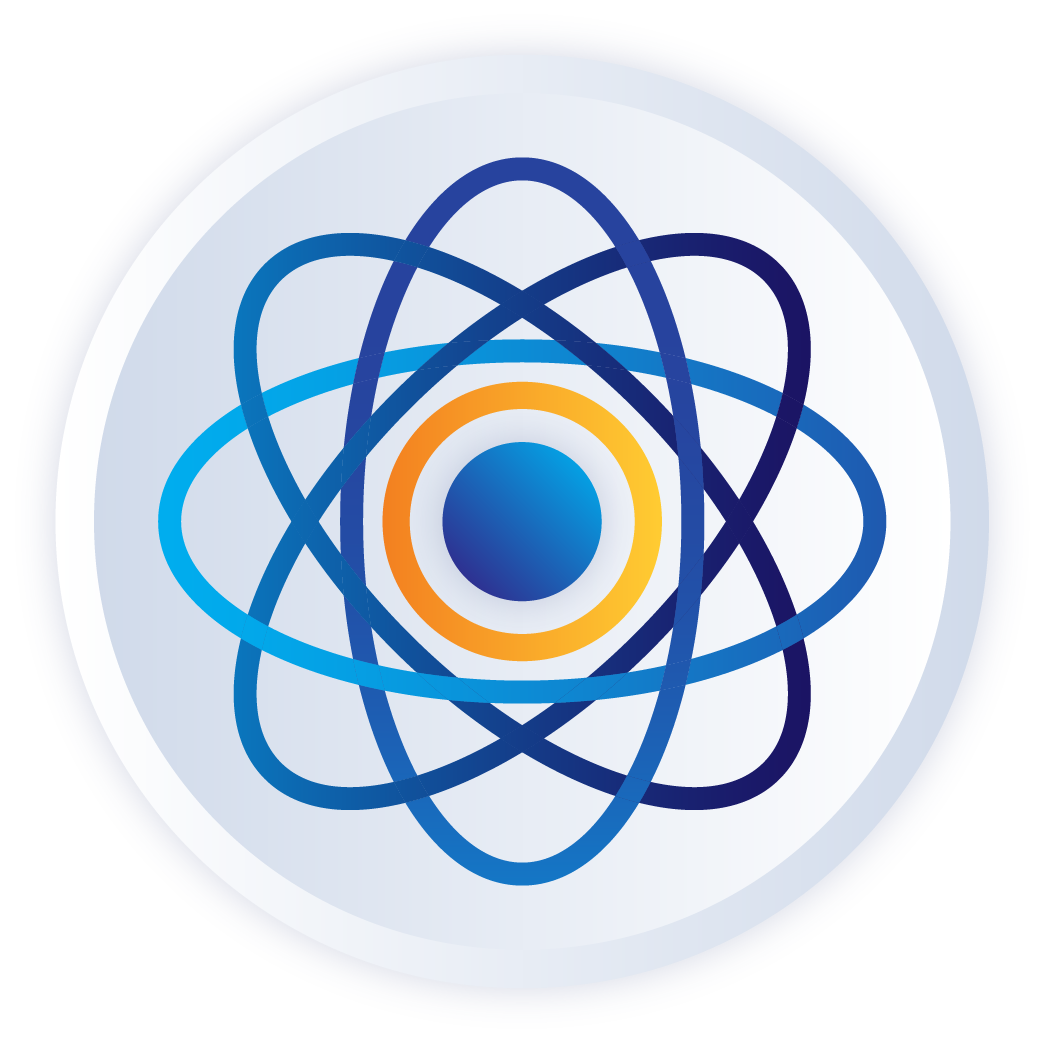Over the last few decades, the word ‘quantum’ has become increasingly popular. It is common to find articles, reports, and many people interested in quantum mechanics and the new capabilities and improvements it brings to the scientific community. This topic not only concerns physics, since the development of quantum mechanics impacts on several other fields such as chemistry, economics, artificial intelligence, operations research, and undoubtedly, cryptography.

This post begins a trio of blogs describing the impact of quantum computing on cryptography, and how to use stronger algorithms resistant to the power of quantum computing.

• This post introduces quantum computing and describes the main aspects of this new computing model and its devastating impact on security standards; it summarizes some approaches to securing information using quantum-resistant algorithms.
• Due to the relevance of this matter, we present our experiments on a large-scale deployment of quantum-resistant algorithms.
• Our third post introduces CIRCL, open-source Go library featuring optimized implementations of quantum-resistant algorithms and elliptic curve-based primitives.

All of this is part of Cloudflare’s Crypto Week 2019, now fasten your seatbelt and get ready to make a quantum leap.

### What is Quantum Computing?

Back in 1981, Richard Feynman raised the question about what kind of computers can be used to simulate physics. However, some physical phenomena, such as quantum mechanics, cannot be simulated using a classical computer. Then, he conjectured the existence of a computer model that behaves under quantum mechanics rules, which opened a field of research now called quantum computing. To understand the basics of quantum computing, it is necessary to recall how classical computers work, and from that shine a spotlight on the differences between these computational models.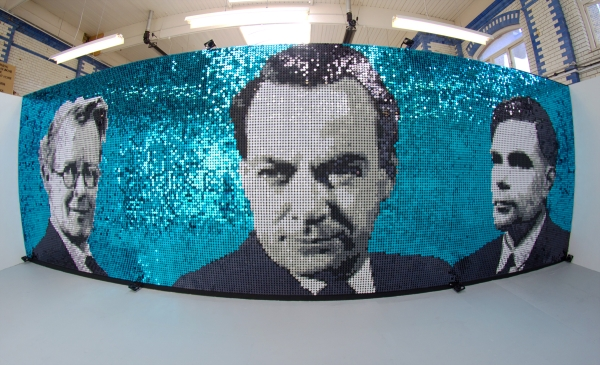Fellows of the Royal Society: John Maynard Smith, Richard Feynman & Alan Turing

In 1936, Alan Turing and Emil Post independently described models that gave rise to the foundation of the computing model known as the Post-Turing machine, which describes how computers work and allowed further determination of limits for solving problems.

In this model, the units of information are bits, which store one of two possible values, usually denoted by 0 and 1. A computing machine contains a set of bits and performs operations that modify the values of the bits, also known as the machine’s state. Thus, a machine with N bits can be in one of 2ᴺ possible states. With this in mind, the Post-Turing computing model can be abstractly described as a machine of states, in which running a program is translated as machine transitions along the set of states.

A paper David Deutsch published in 1985 describes a computing model that extends the capabilities of a Turing machine based on the theory of quantum mechanics. This computing model introduces several advantages over the Turing model for processing large volumes of information. It also presents unique properties that deviate from the way we understand classical computing. Most of these properties come from the nature of quantum mechanics. We’re going to dive into these details before approaching the concept of quantum computing.

### Superposition

One of the most exciting properties of quantum computing that provides an advantage over the classical computing model is superposition. In physics, superposition is the ability to produce valid states from the addition or superposition of several other states that are part of a system.

Applying these concepts to computing information, it means that there is a system in which it is possible to generate a machine state that represents a (weighted) sum of the states 0 and 1; in this case, the term weighted means that the state can keep track of “the quantity of” 0 and 1 present in the state. In the classical computation model, one bit can only store either the state of 0 or 1, not both; even using two bits, they cannot represent the weighted sum of these states. Hence, to make a distinction from the basic states, quantum computing uses the concept of a quantum bit (qubit) -- a unit of information to denote the superposition of two states. This is a cornerstone concept of quantum computing as it provides a way of tracking more than a single state per unit of information, making it a powerful tool for processing information.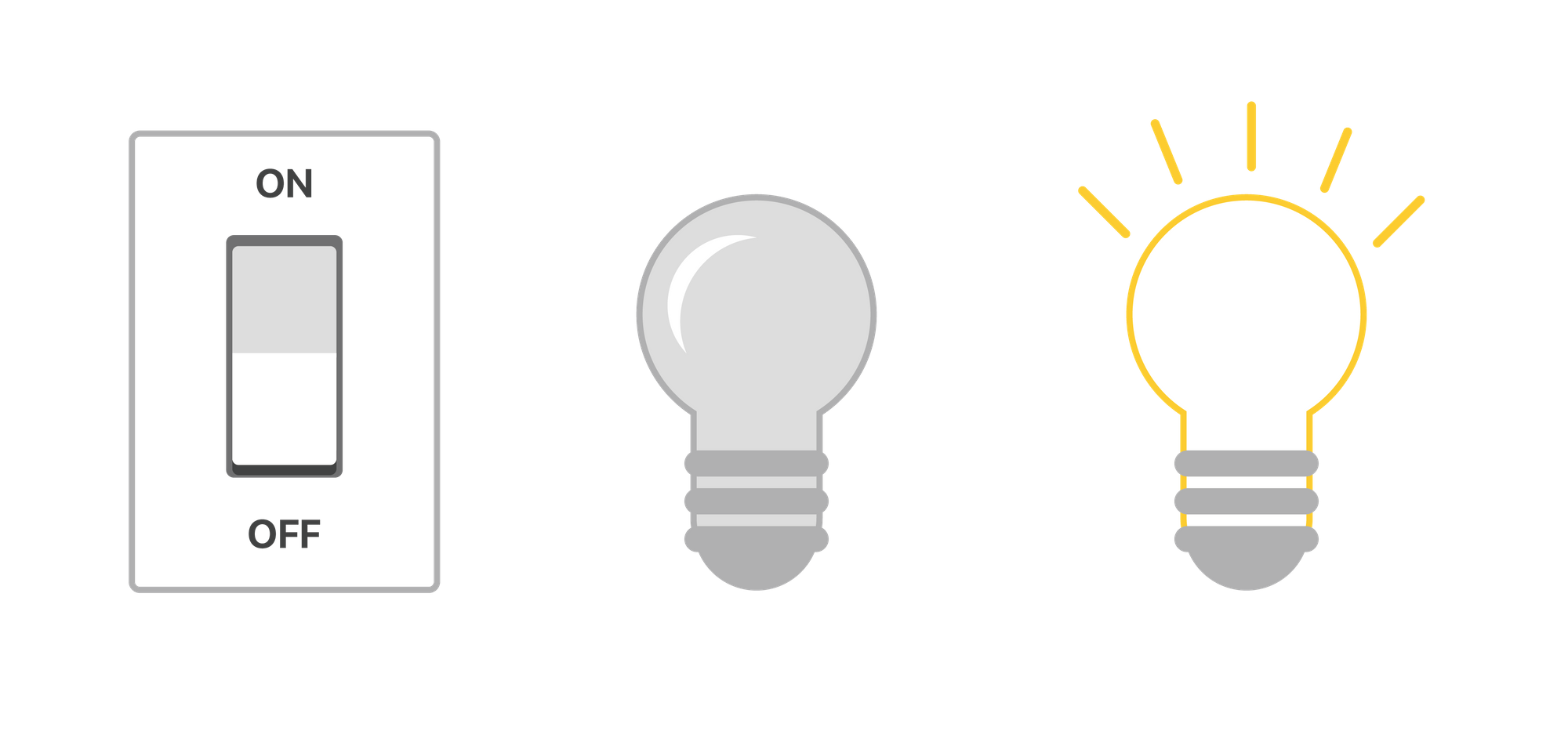Classical computing – A bit stores only one of two possible states: ON or OFF.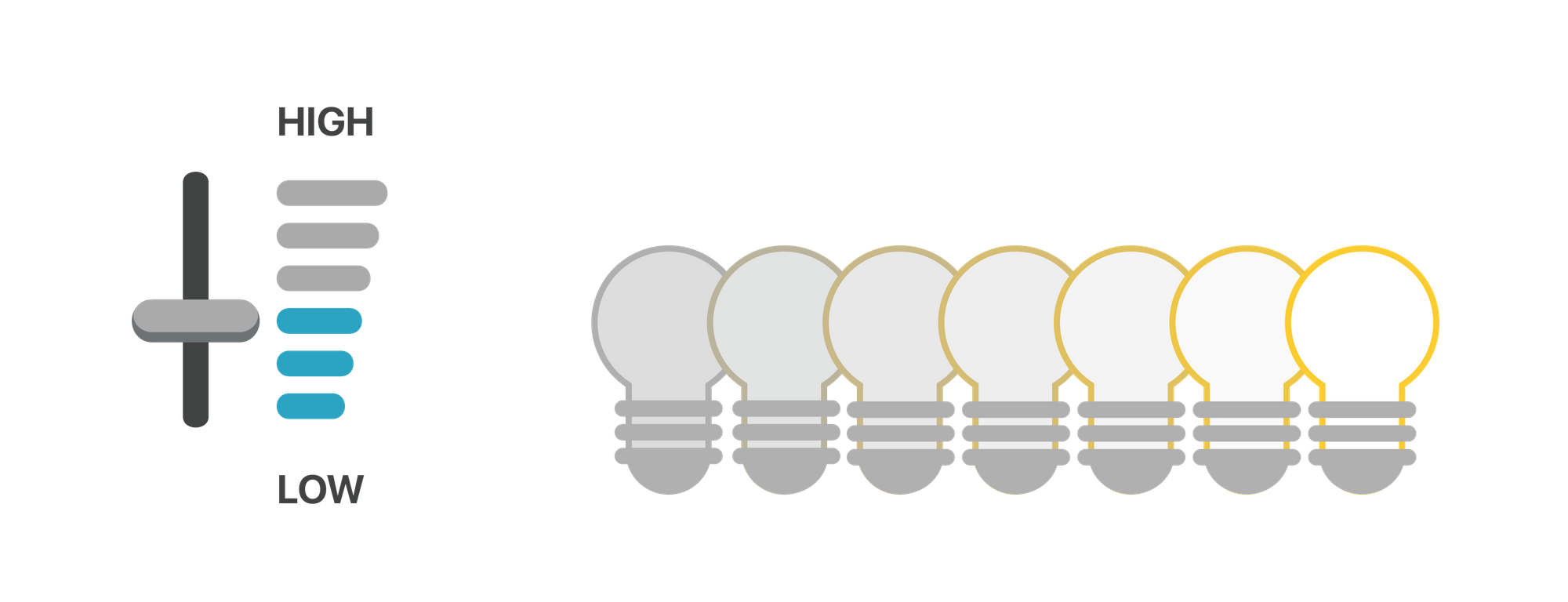Quantum computing – A qubit stores a combination of two or more states.

So, a qubit represents the sum of two parts: the 0 or 1 state plus the amount each 0/1 state contributes to produce the state of the qubit.

In mathematical notation, qubit $$| \Psi \rangle$$ is an explicit sum indicating that a qubit represents the superposition of the states 0 and 1. This is the Dirac notation used to describe the value of a qubit $$| \Psi \rangle = A | 0 \rangle +B | 1 \rangle$$, where, A and B are complex numbers known as the amplitude of the states 0 and 1, respectively. The value of the basic states is represented by qubits as $$| 0 \rangle = 1 | 0 \rangle + 0 | 1 \rangle$$  and $$| 1 \rangle = 0 | 0 \rangle + 1 | 1 \rangle$$, respectively. The right side of the term contains the abbreviated notation for these special states.

### Measurement

In a classical computer, the values 0 and 1 are implemented as digital signals. Measuring the current of the signal automatically reveals the status of a bit. This means that at any moment the value of the bit can be observed or measured.

The state of a qubit is maintained in a physically closed system, meaning that the properties of the system, such as superposition, require no interaction with the environment; otherwise any interaction, like performing a measurement, can cause interference on the state of a qubit.

Measuring a qubit is a probabilistic experiment. The result is a bit of information that depends on the state of the qubit. The bit, obtained by measuring $$| \Psi \rangle = A | 0 \rangle +B | 1 \rangle$$, will be equal to 0 with probability $$|A|^2$$,  and equal to 1 with probability $$|B|^2$$, where $$|x|$$ represents the absolute value of $$x$$.

From Statistics, we know that the sum of probabilities of all possible events is always equal to 1, so it must hold that $$|A|^2 +|B|^2 =1$$. This last equation motivates to represent qubits as the points of a circle of radius one, and more generally, as the points on the surface of a sphere of radius one, which is known as the Bloch Sphere.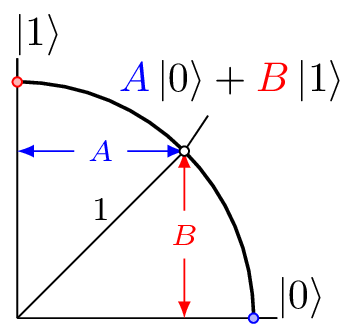The qubit state is analogous to a point on a unitary circle.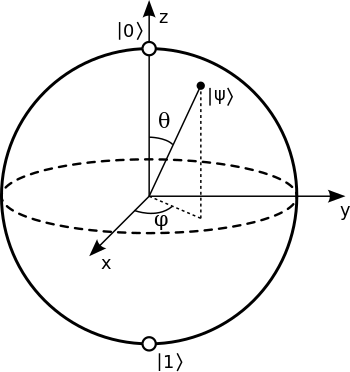The Bloch Sphere by Smite-Meister - Own work, CC BY-SA 3.0.

Let’s break it down: If you measure a qubit you also destroy the superposition of the qubit, resulting in a superposition state collapse, where it assumes one of the basics states, providing your final result.

Another way to think about superposition and measurement is through the coin tossing experiment.

Toss a coin in the air and you give people a random choice between two options: heads or tails. Now, don't focus on the randomness of the experiment, instead note that while the coin is rotating in the air, participants are uncertain which side will face up when the coin lands. Conversely, once the coin stops with a random side facing up, participants are 100% certain of the status.

How does it relate? Qubits are similar to the participants. When a qubit is in a superposition of states, it is tracking the probability of heads or tails, which is the participants’ uncertainty quotient while the coin is in the air. However, once you start to measure the qubit to retrieve its value, the superposition vanishes, and a classical bit value sticks: heads or tails. Measurement is that moment when the coin is static with only one side facing up.

A fair coin is a coin that is not biased. Each side (assume 0=heads and 1=tails) of a fair coin has the same probability of sticking after a measurement is performed. The qubit $$\tfrac{1}{\sqrt{2}}|0\rangle + \tfrac{1}{\sqrt{2}}|1\rangle$$ describes the probabilities of tossing a fair coin. Note that squaring either of the amplitudes results in ½, indicating that there is a 50% chance either heads or tails sticks.

It would be interesting to be able to charge a fair coin at will while it is in the air. Although this is the magic of a professional illusionist, this task, in fact, can be achieved by performing operations over qubits. So, get ready to become the next quantum magician!

### Quantum Gates

A logic gate represents a Boolean function operating over a set of inputs (on the left) and producing an output (on the right). A logic circuit is a set of connected logic gates, a convenient way to represent bit operations.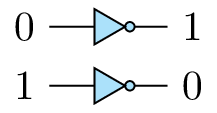The NOT gate is a single-bit operation that flips the value of the input bit.

Other gates are AND, OR, XOR, and NAND, and more. A set of gates is universal if it can generate other gates. For example, NOR and NAND gates are universal since any circuit can be constructed using only these gates.

Quantum computing also admits a description using circuits. Quantum gates operate over qubits, modifying the superposition of the states. For example, there is a quantum gate analogous to the NOT gate, the X gate.

The X quantum gate interchanges the amplitudes of the states of the input qubit.

The Z quantum gate flips the sign’s amplitude of state 1:

Another quantum gate is the Hadamard gate, which generates an equiprobable superposition of the basic states.

Using our coin tossing analogy, the Hadamard gate has the action of tossing a fair coin to the air. In quantum circuits, a triangle represents measuring a qubit, and the resulting bit is indicated by a double-wire.

Other gates, such as the CNOT gate, Pauli’s gates, Toffoli gate, Deutsch gate, are slightly more advanced. Quirk, the open-source playground, is a fun sandbox where you can construct quantum circuits using all of these gates.

### Reversibility

An operation is reversible if there exists another operation that rolls back the output state to the initial state. For instance, a NOT gate is reversible since applying a second NOT gate recovers the initial input.

In contrast, AND, OR, NAND gates are not reversible. This means that some classical computations cannot be reversed by a classic circuit that uses only the output bits. However, if you insert additional bits of information, the operation can be reversed.

Quantum computing mainly focuses on reversible computations, because there’s always a way to construct a reversible circuit to perform an irreversible computation. The reversible version of a circuit could require the use of ancillary qubits as auxiliary (but not temporary) variables.

Due to the nature of composed systems, it could be possible that these ancillas (extra qubits) correlate to qubits of the main computation. This correlation makes it infeasible to reuse ancillas since any modification could have the side-effect on the operation of a reversible circuit. This is like memory assigned to a process by the operating system: the process cannot use memory from other processes or it could cause memory corruption, and processes cannot release their assigned memory to other processes. You could use garbage collection mechanisms for ancillas, but performing reversible computations increases your qubit budget.

### Composed Systems

In quantum mechanics, a single qubit can be described as a single closed system: a system that has no interaction with the environment nor other qubits. Letting qubits interact with others leads to a composed system where more states are represented. The state of a 2-qubit composite system is denoted as $$A_0|00\rangle+A_1|01\rangle+A_2|10\rangle+A_3|11\rangle$$, where, $$A_i$$ values correspond to the amplitudes of the four basic states 00, 01, 10, and 11. This qubit $$\tfrac{1}{2}|00\rangle+\tfrac{1}{2}|01\rangle+\tfrac{1}{2}|10\rangle+\tfrac{1}{2}|11\rangle$$ represents the superposition of these basic states, both having the same probability obtained after measuring the two qubits.

In the classical case, the state of N bits represents only one of 2ᴺ possible states, whereas a composed state of N qubits represents all the 2ᴺ states but in superposition. This is one big difference between these computing models as it carries two important properties: entanglement and quantum parallelism.

### Entanglement

According to the theory behind quantum mechanics, some composed states can be described through the description of its constituents. However, there are composed states where no description is possible, known as entangled states.

The entanglement phenomenon was pointed out by Einstein, Podolsky, and Rosen in the so-called EPR paradox. Suppose there is a composed system of two entangled qubits, in which by performing a measurement in one qubit causes interference in the measurement of the second. This interference occurs even when qubits are separated by a long distance, which means that some information transfer happens faster than the speed of light. This is how quantum entanglement conflicts with the theory of relativity, where information cannot travel faster than the speed of light. The EPR paradox motivated further investigation for deriving new interpretations about quantum mechanics and aiming to resolve the paradox.

Quantum entanglement can help to transfer information at a distance by following a communication protocol. The following protocol examples rely on the fact that Alice and Bob separately possess one of two entangled qubits:

• The superdense coding protocol allows Alice to communicate a 2-bit message $$m_0,m_1$$ to Bob using a quantum communication channel, for example, using fiber optics to transmit photons. All Alice has to do is operate on her qubit according to the value of the message and send the resulting qubit to Bob. Once Bob receives the qubit, he measures both qubits, noting that the collapsed 2-bit state corresponds to Alice’s message.

• The quantum teleportation protocol allows Alice to transmit a qubit to Bob without using a quantum communication channel. Alice measures the qubit to send Bob and her entangled qubit resulting in two bits. Alice sends these bits to Bob, who operates on his entangled qubit according to the bits received and notes that the result state matches the original state of Alice’s qubit.

### Quantum Parallelism

Composed systems of qubits allow representation of more information per composed state. Note that operating on a composed state of N qubits is equivalent to operating over a set of 2ᴺ states in superposition. This procedure is quantum parallelism. In this setting, operating over a large volume of information gives the intuition of performing operations in parallel, like in the parallel computing paradigm; one big caveat is that superposition is not equivalent to parallelism.

Remember that a composed state is a superposition of several states so, a computation that takes a composed state of inputs will result in a composed state of outputs. The main divergence between classical and quantum parallelism is that quantum parallelism can obtain only one of the processed outputs. Observe that a measurement in the output of a composed state causes that the qubits collapse to only one of the outputs, making it unattainable to calculate all computed values.

Although quantum parallelism does not match precisely with the traditional notion of parallel computing, you can still leverage this computational power to get related information.

Deutsch-Jozsa Problem: Assume $$F$$ is a function that takes as input N bits, outputs one bit, and is either constant (always outputs the same value for all inputs) or balanced (outputs 0 for half of the inputs and 1 for the other half). The problem is to determine if $$F$$ is constant or balanced.

The quantum algorithm that solves the Deutsch-Jozsa problem uses quantum parallelism. First, N qubits are initialized in a superposition of 2ᴺ states. Then, in a single shot, it evaluates $$F$$ for all of these states.

The result of applying $$F$$ appears (in the exponent) of the amplitude of the all-zero state. Note that only when $$F$$ is constant is this amplitude, either +1 or -1. If the result of measuring the N qubit is an all-zeros bitstring, then there is a 100% certainty that $$F$$ is constant. Any other result indicates that $$F$$ is balanced.

A deterministic classical algorithm solves this problem using $$2^{N-1}+1$$ evaluations of $$F$$ in the worst case. Meanwhile, the quantum algorithm requires only one evaluation. The Deutsch-Jozsa problem exemplifies the exponential advantage of a quantum algorithm over classical algorithms.

## Quantum Computers

The theory of quantum computing is supported by investigations in the field of quantum mechanics. However, constructing a quantum machine requires a physical system that allows representing qubits and manipulation of states in a reliable and precise way.

The DiVincenzo Criteria require that a physical implementation of a quantum computer must:

1. Be scalable and have well-defined qubits.
2. Be able to initialize qubits to a state.
3. Have long decoherence times to apply quantum error-correcting codes. Decoherence of a qubit happens when the qubit interacts with the environment, for example, when a measurement is performed.
4. Use a universal set of quantum gates.
5. Be able to measure single qubits without modifying others.

Quantum computer physical implementations face huge engineering obstacles to satisfy these requirements. The most important challenge is to guarantee low error rates during computation and measurement. Lowering these rates require techniques for error correction, which add a significant number of qubits specialized on this task. For this reason, the number of qubits of a quantum computer should not be regarded as for classical systems. In a classical computer, the bits of a computer are all effective for performing a calculation, whereas the number of qubits is the sum of the effective qubits (those used to make calculations) plus the ancillas (used for reversible computations) plus the error correction qubits.

Current implementations of quantum computers partially satisfy the DiVincenzo criteria. Quantum adiabatic computers fit in this category since they do not operate using quantum gates. For this reason, they are not considered to be universal quantum computers.

A recurrent problem in optimization is to find the global minimum of an objective function. For example, a route-traffic control system can be modeled as a function that reduces the cost of routing to a minimum. Simulated annealing is a heuristic procedure that provides a good solution to these types of problems. Simulated annealing finds the solution state by slowly introducing changes (the adiabatic process) on the variables that govern the system.

Quantum annealing is the analogous quantum version of simulated annealing. A qubit is initialized into a superposition of states representing all possible solutions to the problem. Here is used the Hamiltonian operator, which is the sum of vectors of potential and kinetic energies of the system. Hence, the objective function is encoded using this operator describing the evolution of the system in correspondence with time. Then, if the system is allowed to evolve very slowly, it will eventually land on a final state representing the optimal value of the objective function.

Currently, there exist adiabatic computers in the market, such as the D-Wave and IBM Q systems, featuring hundreds of qubits; however, their capabilities are somewhat limited to some problems that can be modeled as optimization problems. The limits of adiabatic computers were studied by van Dam et al, showing that despite solving local searching problems and even some instances of the max-SAT problem, there exists harder searching problems this computing model cannot efficiently solve.

### Nuclear Magnetic Resonance

Nuclear Magnetic Resonance (NMR) is a physical phenomena that can be used to represent qubits. The spin of atomic nucleus of molecules is perturbed by an oscillating magnetic field. A 2001 report describes successful implementation of Shor’s algorithm in a 7-qubit NMR quantum computer. An iconic result since this computer was able to factor the number 15.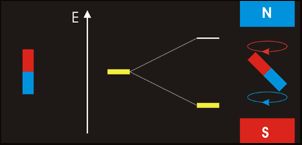Nucleus spinning induced by a magnetic field, Darekk2 - CC BY-SA 3.0

### Superconducting Quantum Computers

One way to physically construct qubits is based on superconductors, materials that conduct electric current with zero resistance when exposed to temperatures close to absolute zero.

The Josephson effect, in which current flows across the junction of two superconductors separated by a non-superconducting material, is used to physically implement a superposition of states.

When a magnetic flux is applied to this junction, the current flows continuously in one direction. But, depending on the quantity of magnetic flux applied, the current can also flow in the opposite direction. There exists a quantum superposition of currents going both clockwise and counterclockwise leading to a physical implementation of a qubit called flux qubit. The complete device is known as the Superconducting Quantum Interference Device (SQUID) and can be easily coupled scaling the number of qubits. Thus, SQUIDs are like the transistors of a quantum computer.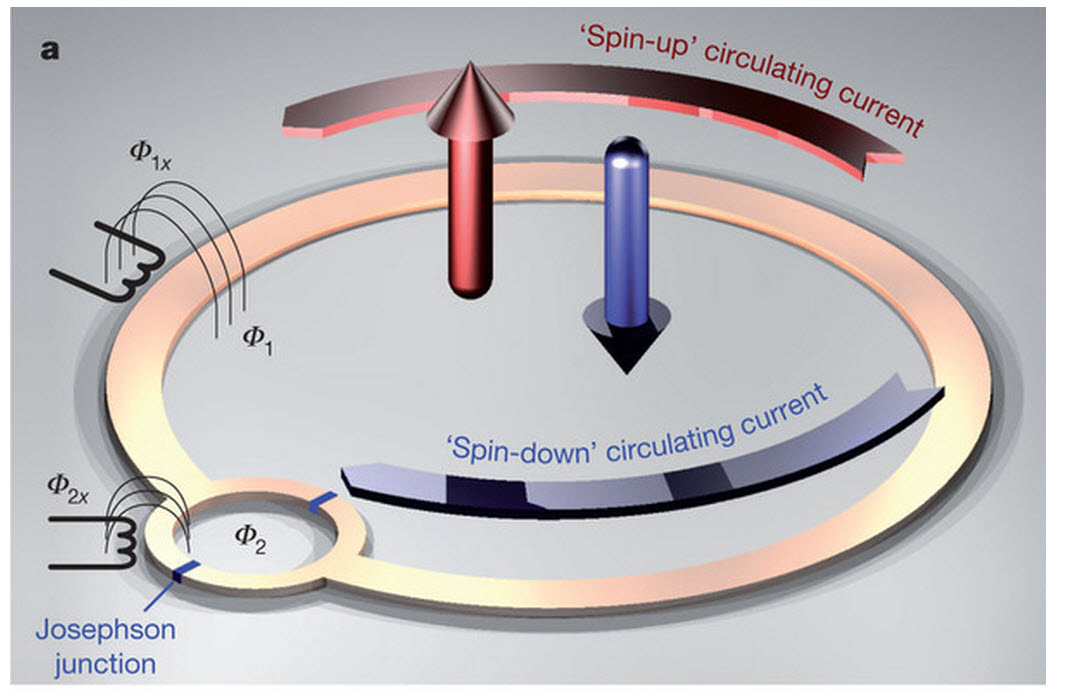SQUID: Superconducting Quantum Interference Device. Image by Kurzweil Network and original source.

Examples of superconducting computers are:

• D-wave’s adiabatic computers process quantum annealing for solving diverse optimization problems.
• Google’s 72-qubit computer was recently announced and also several engineering issues such as achieving lower temperatures.
• IBM’s IBM-Q Tokyo, a 20-qubit adiabatic computer, and IBM Q Experience, a cloud-based system for exploring quantum circuits.

IBM Q System

## The Imminent Threat of Quantum Algorithms

The quantum zoo website tracks problems that can be solved using quantum algorithms. As of mid-2018, more than 60 problems appear on this list, targeting diverse applications in the area of number theory, approximation, simulation, and searching. As terrific as it sounds, some easily-solvable problems by quantum computing are surrounding the security of information.

### Grover’s Algorithm

Tales of a quantum detective (fragment). A couple of detectives have the mission of finding one culprit in a group of suspects that always respond to this question honestly: “are you guilty?”.
The detective C follows a classic interrogative method and interviews every person one at a time, until finding the first one that confesses.
The detective Q proceeds in a different way, First gather all suspects in a completely dark room, and after that, the detective Q asks them -- are you guilty? -- A steady sound comes from the room saying “No!” while at the same time, a single voice mixed in the air responds “Yes!.” Since everybody is submerged in darkness, the detective cannot see the culprit. However, detective Q knows that, as long as the interrogation advances, the culprit will feel desperate and start to speak louder and louder, and so, he continues asking the same question. Suddenly, detective Q turns on the lights, enters into the room, and captures the culprit. How did he do it?

The task of the detective can be modeled as a searching problem. Given a Boolean function $$f$$ that takes N bits and produces one bit, to find the unique input $$x$$ such that $$f(x)=1$$.

A classical algorithm (detective C) finds $$x$$ using $$2^N-1$$ function evaluations in the worst case. However, the quantum algorithm devised by Grover, corresponding to detective Q, searches quadratically faster using around $$2^{N/2}$$ function evaluations.

The key intuition of Grover’s algorithm is increasing the amplitude of the state that represents the solution while maintaining the other states in a lower amplitude. In this way, a system of N qubits, which is a superposition of 2ᴺ possible inputs, can be continuously updated using this intuition until the solution state has an amplitude closer to 1. Hence, after updating the qubits many times, there will be a high probability to measure the solution state.

Initially, a superposition of 2ᴺ states (horizontal axis) is set, each state has an amplitude (vertical axis) close to 0. The qubits are updated so that the amplitude of the solution state increases more than the amplitude of other states. By repeating the update step, the amplitude of the solution state gets closer to 1, which boosts the probability of collapsing to the solution state after measuring.

Grover’s Algorithm (pseudo-code):

1. Prepare an N qubit $$|x\rangle$$ as a uniform superposition of 2ᴺ states.
2. Update the qubits by performing this core operation. $$|x\rangle \mapsto (-1)^{f(x)} |x\rangle$$ The result of $$f(x)$$ only flips the amplitude of the searched state.
3. Negate the N qubit over the average of the amplitudes.
4. Repeat Step 2 and 3 for $$(\tfrac{\pi}{4}) 2^{ N/2}$$ times.
5. Measure the qubit and return the bits obtained.

Alternatively, the second step can be better understood as a conditional statement:

IF f(x) = 1 THEN
Negate the amplitude of the solution state.
ELSE
/* nothing */
ENDIF


Grover’s algorithm considers function $$f$$ a black box, so with slight modifications, the algorithm can also be used to find collisions on the function. This implies that Grover’s algorithm can find a collision using an asymptotically less number of operations than using a brute-force algorithm.

The power of Grover’s algorithm can be turned against cryptographic hash functions. For instance, a quantum computer running Grover’s algorithm could find a collision on SHA256 performing only 2¹²⁸ evaluations of a reversible circuit of SHA256. The natural protection for hash functions is to increase the output size to double. More generally, most of symmetric key encryption algorithms will survive to the power of Grover’s algorithm by doubling the size of keys.

The scenario for public-key algorithms is devastating in face of Peter Shor’s algorithm.

### Shor’s Algorithm

Multiplying integers is an easy task to accomplish, however, finding the factors that compose an integer is difficult. The integer factorization problem is to decompose a given integer number into its prime factors. For example, 42 has three factors 2, 3, and 7 since $$2\times 3\times 7 = 42$$. As the numbers get bigger, integer factorization becomes more difficult to solve, and the hardest instances of integer factorization are when the factors are only two different large primes. Thus, given an integer number $$N$$, to find primes $$p$$ and $$q$$ such that $$N = p \times q$$, is known as integer splitting.

Factoring integers is like cutting wood, and the specific task of splitting integers is analogous to using an axe for splitting the log in two parts. There exist many different tools (algorithms) for accomplishing each task.

For integer factorization, trial division, the Rho method, the elliptic curve method are common algorithms. Fermat's method, the quadratic- and rational-sieve, leads to the (general) number field sieve (NFS) algorithm for integer splitting. The latter relies on finding a congruence of squares, that is, splitting $$N$$ as a product of squares such that $$N = x^2 - y^2 = (x+y)\times(x-y)$$ The complexity of NFS is mainly attached to the number of pairs $$(x, y)$$ that must be examined before getting a pair that factors $$N$$. The NFS algorithm has subexponential complexity on the size of $$N$$, meaning that the time required for splitting an integer increases significantly as the size of $$N$$ grows. For large integers, the problem becomes intractable for classical computers.

### The Axe of Thor Shor

The many different guesses of the NFS algorithm are analogous to hitting the log using a dulled axe; after subexponential many tries, the log is cut by half. However, using a sharper axe allows you to split the log faster. This sharpened axe is the quantum algorithm proposed by Shor in 1994.

Let $$x$$ be an integer less than $$N$$ and of the order $$k$$. Then, if $$k$$ is even, there exists an integer $$q$$ so $$qN$$ can be factored as follows.

This approach has some issues. For example, the factorization could correspond to $$q$$ not $$N$$ and the order of $$x$$ is unknown, and here is where Shor’s algorithm enters the picture, finding the order of $$x$$.

The internals of Shor’s algorithm rely on encoding the order $$k$$ into a periodic function, so that its period can be obtained using the quantum version of the Fourier transform (QFT). The order of $$x$$ can be found using a polynomial number quantum evaluations of Shor’s algorithm. Therefore, splitting integers using this quantum approach has polynomial complexity on the size of $$N$$.

Shor’s algorithm carries strong implications on the security of the RSA encryption scheme because its security relies on integer factorization. A large-enough quantum computer can efficiently break RSA for current instances.

Alternatively, one may recur to elliptic curves, used in cryptographic protocols like ECDSA or ECDH. Moreover, all TLS ciphersuites use a combination of elliptic curve groups, large prime groups, and RSA and DSA signatures. Unfortunately, these algorithms all succumb to Shor’s algorithm. It only takes a few modifications for Shor’s algorithm to solve the discrete logarithm problem on finite groups. This sounds like a catastrophic story where all of our encrypted data and privacy are no longer secure with the advent of a quantum computer, and in some sense this is true.

On one hand, it is a fact that the quantum computers constructed as of 2019 are not large enough to run, for instance, Shor’s algorithm for the RSA key sizes used in standard protocols. For example, a 2018 report shows experiments on the factorization of a 19-bit number using 94 qubits, they also estimate that 147456 qubits are needed for factoring a 768-bit number. Hence, there numbers indicates that we are still far from breaking RSA.

What if we increment RSA key sizes to be resistant to quantum algorithms, just like for symmetric algorithms?

Bernstein et al. estimated that RSA public keys should be as large as 1 terabyte to maintain secure RSA even in the presence of quantum factoring algorithms. So, for public-key algorithms, increasing the size of keys does not help.

A recent investigation by Gidney and Ekerá shows improvements that accelerate the evaluation of quantum factorization. In their report, the cost of factoring 2048-bit integers is estimated to take a few hours using a quantum machine of 20 million qubits, which is far from any current development. Something worth noting is that the number of qubits needed is two orders of magnitude smaller than the estimated numbers given in previous works developed in this decade. Under these estimates, current encryption algorithms will remain secure several more years; however, consider the following not-so-unrealistic situation.

Information currently encrypted with for example, RSA, can be easily decrypted with a quantum computer in the future. Now, suppose that someone records encrypted information and stores them until a quantum computer is able to decrypt ciphertexts. Although this could be as far as 20 years from now, the forward-secrecy principle is violated. A 20-year gap to the future is sometimes difficult to imagine. So, let’s think backwards, what would happen if all you did on the Internet at the end of the 1990s can be revealed 20 years later -- today. How does this impact the security of your personal information? What if the ciphertexts were company secrets or business deals? In 1999, most of us were concerned about the effects of the Y2K problem, now we’re facing Y2Q (years to quantum): the advent of quantum computers.

### Post-Quantum Cryptography

Although the current capacity of the physical implementation of quantum computers is far from a real threat to secure communications, a transition to use stronger problems to protect information has already started. This wave emerged as post-quantum cryptography (PQC). The core idea of PQC is finding algorithms difficult enough that no quantum (and classical) algorithm can solve them.

A recurrent question is: How does it look like a problem that even a quantum computer can not solve?

These so-called quantum-resistant algorithms rely on different hard mathematical assumptions; some of them as old as RSA, others more recently proposed. For example, McEliece cryptosystem, formulated in the late 70s, relies on the hardness of decoding a linear code (in the sense of coding theory). The practical use of this cryptosystem didn’t become widespread, since with the passing of time, other cryptosystems superseded in efficiency. Fortunately, McEliece cryptosystem remains immune to Shor’s algorithm, gaining it relevance in the post-quantum era.

Post-quantum cryptography presents alternatives:

As of 2017, the NIST started an evaluation process that tracks possible alternatives for next-generation secure algorithms. From a practical perspective, all candidates present different trade-offs in implementation and usage. The time and space requirements are diverse; at this moment, it’s too early to define which will succeed RSA and elliptic curves. An initial round collected 70 algorithms for deploying key encapsulation mechanisms and digital signatures. As of early 2019, 28 of these survive and are currently in the analysis, investigation, and experimentation phase.

Cloudflare's mission is to help build a better Internet. As a proactive action, our cryptography team is preparing experiments on the deployment of post-quantum algorithms at Cloudflare scale. Watch our blog post for more details.